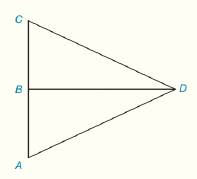Chapter 3.CR, Problem 6CR### Elementary Geometry for College St...

6th Edition
Daniel C. Alexander + 1 other
ISBN: 9781285195698

#### Solutions

Chapter
Section### Elementary Geometry for College St...

6th Edition
Daniel C. Alexander + 1 other
ISBN: 9781285195698
Textbook Problem
9 views

# Given: B is the midpoint of A C ¯ B D ¯ ⊥ A C ¯ Prove: Δ A D C is isoscelesTo determine

To prove:

Explanation

Given:

The given figure is,

Figure (1)

From figure (1).

B is the midpoint of AC¯

BD¯AC¯

Definition:

When the triangle has two of its side length same, then the triangle is called isosceles triangle.

Approach:

The congruent triangles have their corresponding sides and angles congruent.

Consider the triangles,

ΔABD and ΔBCD

From the given data,

The point B is the midpoint of AC¯, then the side BC¯ and the side AB¯ are equal to each other. So,

AB¯BC¯

And, the line BD¯ is perpendicular to the line AC¯.

Then, the angle mCBD and the angle mABD are right angles

### Still sussing out bartleby?

Check out a sample textbook solution.

See a sample solution

#### The Solution to Your Study Problems

Bartleby provides explanations to thousands of textbook problems written by our experts, many with advanced degrees!

Get Started